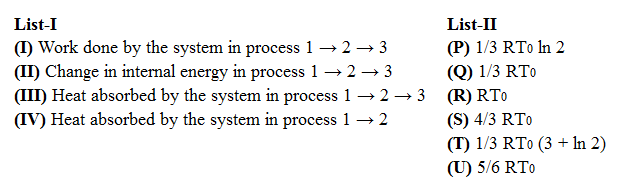### JEE (Advanced) 2019 Paper-2 Question 18

Instructions

In a thermodynamic process on an ideal monatomic gas, the infinitesimal heat absorbed by the gas is given by T $$\triangle X,$$ where T is temperature of the system and $$\triangle X$$ is the infinitesimal change in a thermodynamic quantity X of the system. For a mole of monatomic ideal gas $$X = \frac{3}{2} R ln \left(\frac {T}{T_A}\right) + R ln \left(\frac {V}{V_A}\right)$$ Here, R is gas constant, V is volume of gas, $$T_A$$ and $$V_A$$ are constants.
The List-I below gives some quantities involved in a process and List-II gives some possible values of these quantities.Question 18